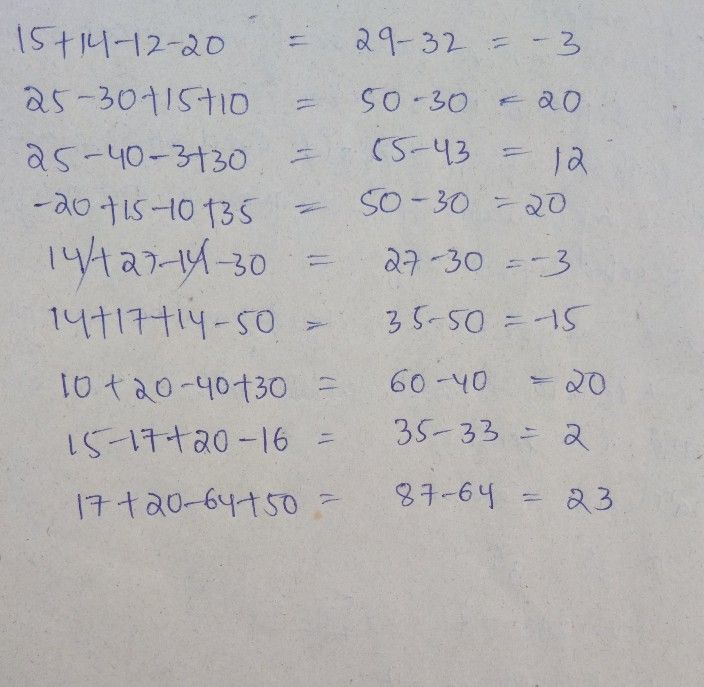Symbol
Problem$-4$ $1$ Mome Gandn En115h $cAc\right)w$ $rt\right)$ Solve:- $25-40-3+30$ $15+14$ $12.21\right)$ $25$ $3$ $+15+10$ $14+17+14-50$ $-$ $20+15.10+35$ $14+27-14-30$ $---$ $17+20-54+50$ $1\bar{0} +20.40+30$ $15.17+29-15$
1st-6th grade
Other
SolutionQanda teacher - draksha